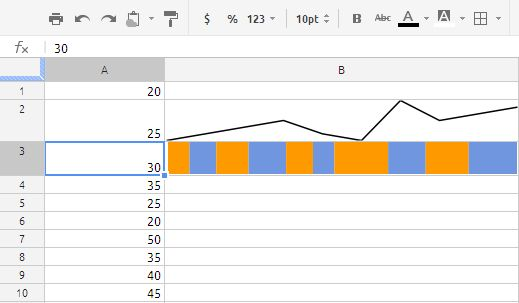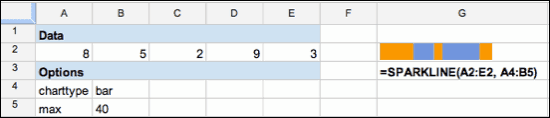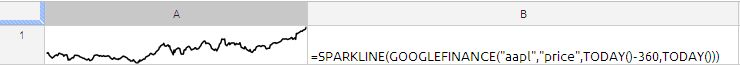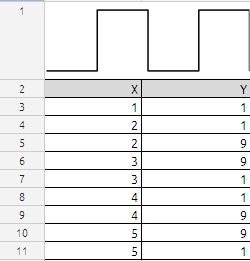# SPARKLINE CHARTS

A sparkline chart is data-intense, design-simple, cell-sized graphics

Syntax of the function is as follows:

SPARKLINE(data, [options])

data: the range of cells or array of data to plot on the chart

options: A range or array of optional settings with their associated values used to

customize the chart

Options:
A) charttype: the type of chart to plot (default is “line”)

1. Use “line” for a line chart

2. Use “bar” for a stacked bar chart

B) For line charts only:

1. xmin: sets the minimum value along the horizontal axis; any number is a valid value

2. xmax: sets the maximum value along the horizontal axis; any number is a valid value

3. ymin: sets the minimum value along the vertical axis; any number is a valid value

4. ymax: sets the maximum value along the vertical axis; any number is a valid value

C) For bar charts only:

1. max: sets the maximum value along the horizontal axis; any number is a valid value

Notes on sparkline syntax

• For line charts, “data” can either be a 1-dimensional array of values or a 2-dimensional array containing values and spacing along the horizontal axis.
• For bar charts, only one row or column of data can be plotted.
• The “text color” and “text background color” buttons in the spreadsheet toolbar can be used to set the color and background color for line charts.
• Each sparkline function only accepts a few hundred data points. If a broken image icon is shown in the cell, try reducing the number of data points plotted on the chart and try again.

Lets see some examples:in cells A1:A10 we have random number between 20 to 50

the formula in cell B2 is
=SPARKLINE(A1:A10)
and the formula in cell B3 is
=SPARKLINE(A1:A10,{“charttype”,”bar”})

From the above it is understood that if we leave the options we will get only line chart,
if we want to prepare the barchart we have to use the options argument.

=SPARKLINE(A2:E2, A4:B5)in the above example we have to observe one important thing:
the options are in two row height and two column width. It has to be like that otherwise the function does not work because if you observe the formula without referring to cells it will be like this

=SPARKLINE(A2:E2,{“charttype”,”bar”;“ymax”,10})

observe semi-colon between “bar” and “ymax” here semi-colon means it is two dimensional array you can add as many options as require by following the above principle.

Plot the price of a stock over a 360-day period for apple is as follows:

=SPARKLINE(GOOGLEFINANCE(“aapl”,”price”,TODAY()-30,TODAY()))Similarly we can generate the Square line charts, Sinwave charts, SawTooth wave chartsformula in cell A1 is

=SPARKLINE(A3:B11, {“charttype”,”line”;”ymax”,10;”ymin”,0} )

1.karl says: